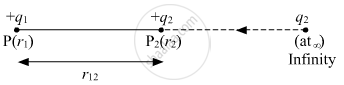# Two Point Charges Q1 and Q2 Are Kept at a Distance of R12 in Air. Deduce the Expression for the Electrostatic Potential Energy of this System. - Physics

Short Note

Two-point charges q1 and q2 are kept at a distance of r12 in air. Deduce the expression for the electrostatic potential energy of this system.

#### Solution

Electrostatic potential energy of a system is defined as the total amount of the work done in bringing the various charges to their respective position from infinitely large mutual separation.

Let us consider a charge q1 at a postion vector r1 and q2 at infinity which is to be brought at point P2 having position vector r2. and dW is the small amount of work done in moving a charge to a distance dx.dW = vec"F". vecdx

dW = -(kq_1q_2)/(r^2). dx

W = -int_∞^(r12) (kq_1q_2)/(r^2).dx

W = -Kq_1q_2 int_∞^(r12) 1/r^2. dx

W = -Kq_1q_2 [-1/r]_∞^(r12)

W = Kq_1q_2[1/(r_12)]

W = U = (Kq_1q_2)/(r_12).

Concept: Relation Between Electric Field and Electrostatic Potential
Is there an error in this question or solution?